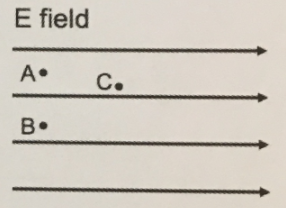# Problem: The diagram shows three spatial points (A, B, and C) in a uniform electric field that points from left to right in the plane of this page. Each point has an associated electric potential (VA, VB, VC). Rank the potentials for these three points, from least to greatest. Add a point D to this diagram such that it is at a lower potential than points A or B but at a higher potential than point C.

###### FREE Expert Solution

Electric potential is defined as the work done against the electric field to bring a charge from infinity to a point within the electric field.

The electric field lines are all going to infinity.

99% (447 ratings)###### Problem Details

The diagram shows three spatial points (A, B, and C) in a uniform electric field that points from left to right in the plane of this page. Each point has an associated electric potential (VA, VB, VC). Rank the potentials for these three points, from least to greatest. Add a point D to this diagram such that it is at a lower potential than points A or B but at a higher potential than point C.Frequently Asked Questions

What scientific concept do you need to know in order to solve this problem?

Our tutors have indicated that to solve this problem you will need to apply the Electric Potential concept. You can view video lessons to learn Electric Potential. Or if you need more Electric Potential practice, you can also practice Electric Potential practice problems.